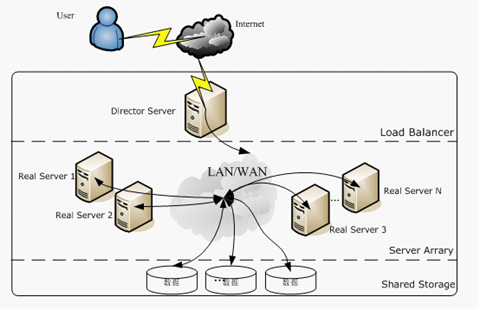# 排序优化算法Learning to Ranking

## Learning to Ranking简介

Learning to Rank （LTR）是指一系列基于机器学习的排序算法，最初主要应用于信息检索（Information Retrieval，IR）领域，最典型的是解决搜索引擎对搜索结果的排序问题。除了信息检索以外，Learning to Rank 也被应用到许多其他排序问题上，如商品推荐、计算广告、生物信息学等。

• 输入空间（input space），以特征向量来表示的样本，特征是以某种方式提取出来的。
• 假设空间（hypothesis space），它定义了一系列从input space 到output space的映射函数。
• 损失函数（loss function），ML的目的就是在training set上定义一个loss function来学习一个optimal hypothesis。loss function在ML中扮演了一个非常关键的角色。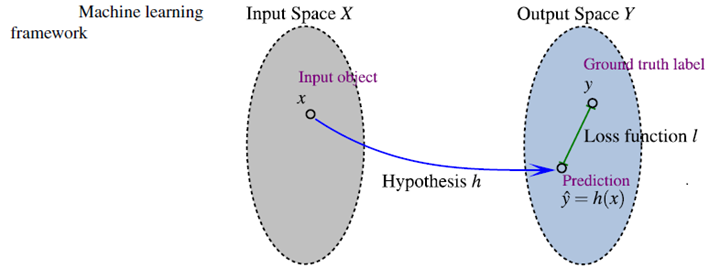LTR的定义：

• Feature Based，样本以特征向量的形式体现。
• Discriminative Training，(有识别性的训练)a learning-to-rank method has its own input space, output space, hypothesis space, and loss function.Discriminative Training是一个基于训练集的自动学习过程。

LTR框架

LTR是有监督学习，因此是需要有标注的training set。很多机器学习都可以套在上述流程图中。不同的方法主要体现在不同的input space，不同的的output space，不同的假设空间，不同的损失函数。经典Learning to Rank框架如下：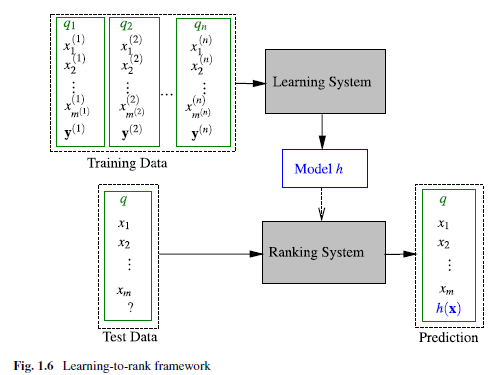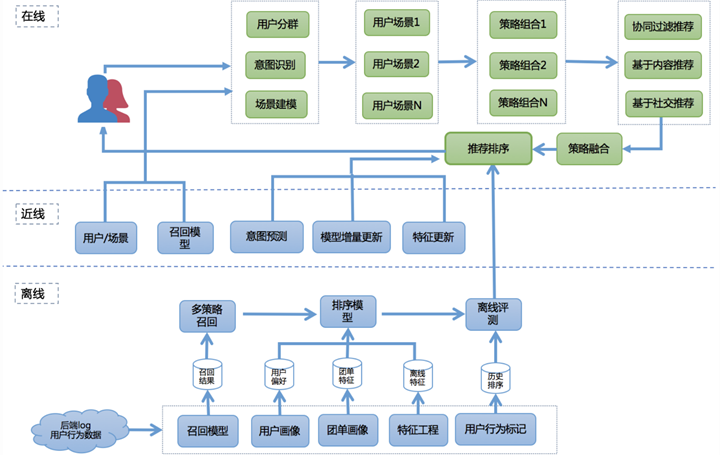LTR的优点

LTR 则是基于特征，通过机器学习算法训练来学习到最佳的拟合公式，相比传统的排序方法，优势有很多：

• 可以根据反馈自动学习并调整参数
• 可以融合多方面的排序影响因素
• 避免过拟合（通过正则项）
• 实现个性化需求（推荐）
• 多种召回策略的融合排序推荐（推荐）
• 多目标学习（推荐）

LTR的局限性

• 在一个机器学习的系统当中，人们很难理解为什么一个结果比另一个结果要好。机器学习系统像一个黑盒子，大部分时候告诉我们结果1比结果2更加相关的概率，但不会告诉我们是因为什么原因结果1比结果2要好。
• 很多information retrieval当中发现的特征很难在机器学习模型中产生效果。因为这些特征常常是针对某一类检索问题，然而对于那一类检索问题，常见的机器学习算法可能会为了模型的概括性以及防止overfitting，忽略特定的特征。这也是为什么当有了足够多的ranking engineer，大部分人都会专注改进rule-based scoring方法，去直接针对特定问题进行改进。

## Learning to Rank 算法分类

### 单点法（Pointwise）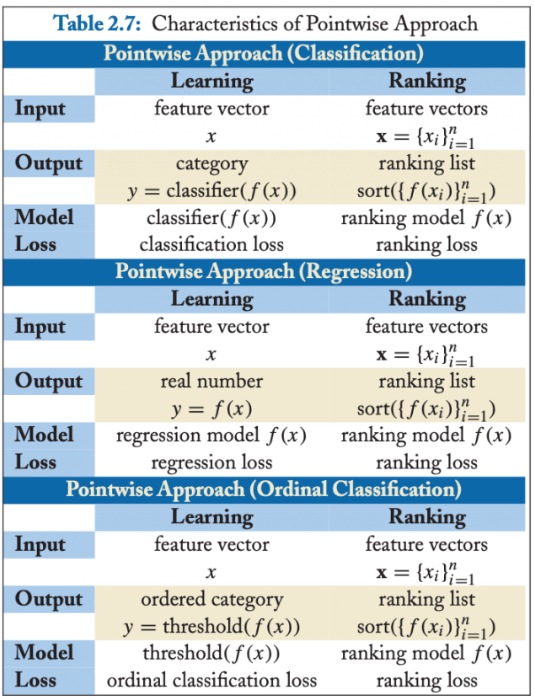• 分类（Classification）：输出空间包含的是无序类别，对每个查询-文档对的样本判断是否相关，可以是二分类的，如相关认为是正例，不相关认为是负例；也可以是类似 NDCG 那样的五级标注的多分类问题。分类模型通常会输出一个概率值，可根据概率值的排序作为排序最终结果。
• 回归（Regression）：输出空间包含的是真实值相关度得分，可通过回归来直接拟合相关度打分。
• 有序分类（Ordinal Classification）：有序分类也称有序回归（Ordinal Regression），输出空间一般包含的是有序类别，通常的做法是找到一个打分函数，然后用一系列阈值对得分进行分割，得到有序类别。

#### 单点法（Pointwise）的应用

• 基于神经网络的排序算法 RankProp
• 基于感知机的在线排序算法 Prank(Perception Rank)/OAP-BPM
• 基于 SVM 的排序算法
• Subset Ranking
• McRank
• Prank
• OC SVM

#### 单点法（Pointwise）的缺点

Pointwise 方法通过优化损失函数求解最优的参数，可以看到 Pointwise 方法非常简单，工程上也易实现，但是 Pointwise 也存在很多问题：

• Pointwise 只考虑单个文档同 query 的相关性，没有考虑文档间的关系，然而排序追求的是排序结果，并不要求精确打分，只要有相对打分即可；
• 通过分类只是把不同的文档做了一个简单的区分，同一个类别里的文档则无法深入区别，虽然我们可以根据预测的概率来区别，但实际上，这个概率只是准确度概率，并不是真正的排序靠前的预测概率；
• Pointwise 方法并没有考虑同一个 query 对应的文档间的内部依赖性。一方面，导致输入空间内的样本不是 IID 的，违反了 ML 的基本假设，另一方面，没有充分利用这种样本间的结构性。其次，当不同 query 对应不同数量的文档时，整体 loss 将容易被对应文档数量大的 query 组所支配，应该每组 query 都是等价的才合理。
• 很多时候，排序结果的 Top N 条的顺序重要性远比剩下全部顺序重要性要高，因为损失函数没有相对排序位置信息，这样会使损失函数可能无意的过多强调那些不重要的 docs，即那些排序在后面对用户体验影响小的 doc，所以对于位置靠前但是排序错误的文档应该加大惩罚。

### 配对法（Pairwise）

• 我们可以针对某一个关键字得到一个完美的排序关系。在实际操作中，这个关系可以通过相关标签来获得，也可以通过其他信息获得，比如点击率等信息。然而，这个完美的排序关系并不是永远都存在的。试想在电子商务网站中，对于查询关键字 “哈利波特”，有的用户希望购买书籍，有的用户则希望购买含有哈利波特图案的 T 恤，显然，这里面就不存在一个完美排序。
• 我们寄希望能够学习文档之间的两两配对关系从而 “重构” 这个完美排序。然而，这也不是一个有 “保证” 的思路。用刚才的例子，希望学习两两关系 “B>C”、“B>A” 和 “C>A” 来重构完美排序 “B>C>A”。然而，实际中，这三个两两关系之间是独立的。特别是在预测的时候，即使模型能够正确判断 “B>C” 和 “C>A”，也不代表模型就一定能得到 “B>A”。注意，这里的关键是 “一定”，也就是模型有可能得到也有可能得不到。两两配对关系不能 “一定” 得到完美排序，这个结论其实就揭示了这种方法的不一致性。也就是说，我们并不能真正保证可以得到最优的排序。
• 我们能够构建样本来描述这样的两两相对的比较关系。一个相对比较简单的情况，认为文档之间的两两关系来自于文档特征（Feature）之间的差异。也就是说，可以利用样本之间特征的差值当做新的特征，从而学习到差值到相关性差异这样的一组对应关系。

Pairwise 最终的算分，分类和回归都可以实现，不过最常用的还是二元分类，如下图：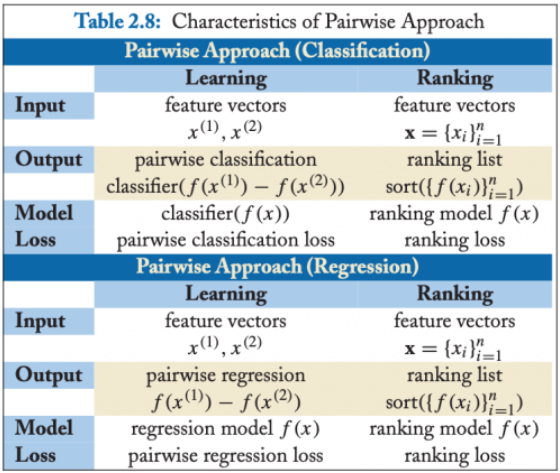#### 配对法（Pairwise）的应用

• 基于 SVM 的 Ranking SVM 算法
• 基于神经网络的 RankNet 算法（2007）
• 基于 Boosting 的 RankBoost 算法（2003）

#### 配对法（Pairwise）的缺点

Pairwise 方法通过考虑两两文档之间的相关对顺序来进行排序，相比 Pointwise 方法有明显改善。但 Pairwise 方法仍有如下问题：

• 使用的是两文档之间相关度的损失函数，而它和真正衡量排序效果的指标之间存在很大不同，甚至可能是负相关的，如可能出现 Pairwise Loss 越来越低，但 NDCG 分数也越来越低的现象。
• 只考虑了两个文档的先后顺序，且没有考虑文档在搜索列表中出现的位置，导致最终排序效果并不理想。
• 不同的查询，其相关文档数量差异很大，转换为文档对之后，有的查询可能有几百对文档，有的可能只有几十个，这样不加均一化地在一起学习，模型会优先考虑文档对数量多的查询，减少这些查询的 loss，最终对机器学习的效果评价造成困难。
• Pairwise 方法的训练样例是偏序文档对，它将对文档的排序转化为对不同文档与查询相关性大小关系的预测；因此，如果因某个文档相关性被预测错误，或文档对的两个文档相关性均被预测错误，则会影响与之关联的其它文档，进而引起连锁反应并影响最终排序结果。

### 列表法（Listwise）

Listwise方法是直接优化排序列表，输入为单条样本为一个文档排列。相对于尝试学习每一个样本是否相关或者两个文档的相对比较关系，列表法排序学习的基本思路是尝试直接优化像 NDCG（Normalized Discounted Cumulative Gain）这样的指标，从而能够学习到最佳排序结果。列表法的相关研究有很大一部分来自于微软研究院。列表法排序学习有两种基本思路。第一种称为 Measure-specific，就是直接针对 NDCG 这样的指标进行优化。目的简单明了，用什么做衡量标准，就优化什么目标。第二种称为 Non-measure specific，则是根据一个已经知道的最优排序，尝试重建这个顺序，然后来衡量这中间的差异。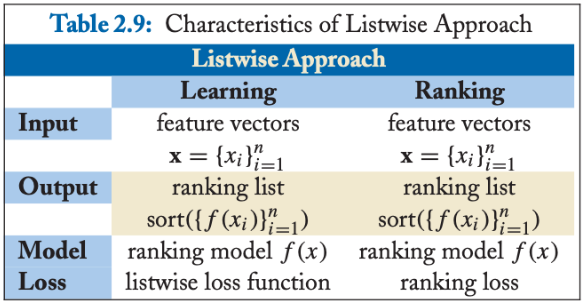1）Measure-specific，直接针对 NDCG 类的排序指标进行优化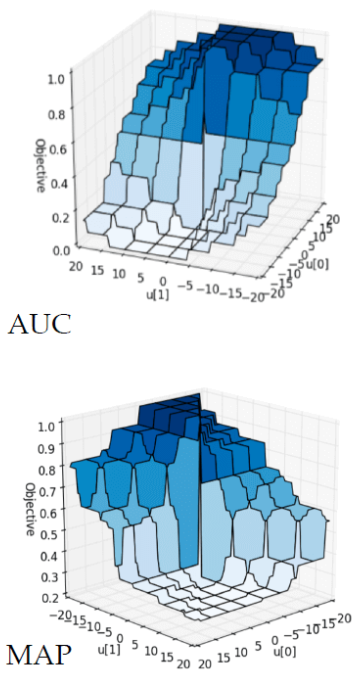• 既然直接优化有难度，那就找一个近似 NDCG 的另外一种指标。而这种替代的指标是 “连续” 和 “可微分” 的 。只要我们建立这个替代指标和 NDCG 之间的近似关系，那么就能够通过优化这个替代指标达到逼近优化 NDCG 的目的。这类的代表性算法的有 SoftRank 和 AppRank。
• 尝试从数学的形式上写出一个 NDCG 等指标的 “边界”（Bound），然后优化这个边界。比如，如果推导出一个上界，那就可以通过最小化这个上界来优化 NDCG。这类的代表性算法有 SVM-MAP 和 SVM-NDCG。
• 希望从优化算法上下手，看是否能够设计出复杂的优化算法来达到优化 NDCG 等指标的目的。对于这类算法来说，算法要求的目标函数可以是 “非连续” 和 “非可微分” 的。这类的代表性算法有 AdaRank 和 RankGP。

2）Non-measure specific，尝试重建最优顺序，衡量其中差异

3）列表法和配对法的中间解法

#### 列表法（Listwise）的应用

• 基于 Measure-specific 的 SoftRank、SVM-MAP、SoftRank、LambdaRank、LambdaMART
• 基于 Non-measure specific 的 ListNet、ListMLE、BoltzRank。

#### 列表法（Listwise）的缺点

• 一些算法需要基于排列来计算 loss，从而使得训练复杂度较高，如 ListNet 和 BoltzRank。
• 位置信息并没有在 loss 中得到充分利用，可以考虑在 ListNet 和 ListMLE 的 loss 中引入位置折扣因子。

## Learning to Ranking的评估指标

### P@K

Precision at K 通常表示为 P@K， 表示 top-k 的结果中有相关结果所占比例，其中 K 表示前 K 位，定义为：

$$Precision@K=\frac{relevant-documents-num-among-top-k}{K}$$

• Prec@3 = 2/3
• Prec@4 = 2/4
• Prec@5 = 3/5

### MAP(Mean Average Precision)

$$AP = \sum_{\alpha =0.01}^{1.0}P_{\alpha}$$

AP衡量的是我们训练得到的模型在每个类别上的好坏，MAP衡量的是该模型在所有类别上的好坏，得到AP后，MAP的计算就变得很简单了，就是取所有AP的平均值。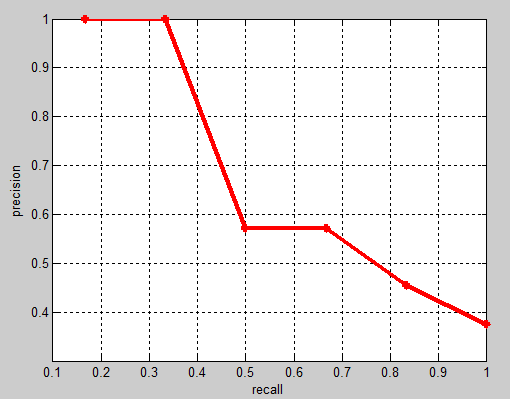$$AP = \int_{0}^{1}\max(\{P(r)|r>=R\})dR$$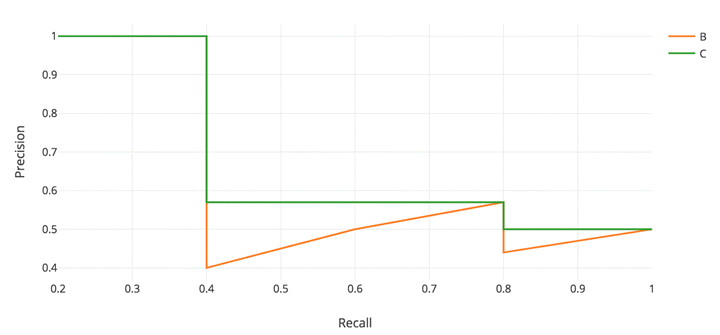$$AP=\frac{\sum_{j=1}^{n_i} P(j) \cdot y_{i,j} }{\sum_{j=1}^{n_i}y_{i,j} }$$

$y_{ij}$即每个doc的label（1和0），而每个query-doc pair的P值代表了到$d_{ij}$这个doc所在的位置为止的precision：

$$P(j)=\frac{\sum_{k:\pi_i(k)\leq \pi_i(j)}y_{i,k} }{\pi_i(j)}$$

MAP 优点

• 给出了一个代表精确度 — 召回率曲线下复杂区域的单一度量。这提供了每个列表的平均精度。
• 处理列表推荐物品的自然排序。这与将检索项视为集合的度量标准形成了对比。
• 这一指标能够给予发生在排序高的推荐名单中的错误更多的权重。相反，它对发生在推荐列表中较深位置的错误的权重较小。这符合在推荐列表的最前面显示尽可能多的相关条目的需要。

MAP 缺点

• 这个度量标准适用于二进制(相关/非相关)评级。然而，它不适合细粒度的数字评级。此度量无法从此信息中提取误差度量。
• 对于细粒度的评分，例如从 1 星到 5 星的评分，评估首先需要对评分进行阈值，以产生二元相关性。一种选择是只考虑大于 4 的评级。由于人工阈值的存在，这在评估度量中引入了偏差。此外，我们正在丢弃那些精细的信息。这个信息是在 4 星和 5 星之间的差异评级，以及在不相关的项目的信息。1 星评级真的和 3 星评级一样吗？

### NDCG(Normalized Discounted Cumulative Gain)

NDCG，Normalized Discounted cumulative gain 直接翻译为归一化折损累计增益。这个指标通常是用来衡量和评价搜索结果算法。DCG的两个思想：

• 高关联度的结果比一般关联度的结果更影响最终的指标得分
• 有高关联度的结果出现在更靠前的位置的时候，指标会越高

CG，cumulative gain，是DCG的前身，只考虑到了相关性的关联程度，没有考虑到位置的因素。它是一个搜素结果相关性分数的总和。指定位置p上的CG为：

$$CG_P=\sum_{i=1}^{p}rel_i$$

$rel_i$代表i这个位置上的相关度。

DCG， Discounted 的CG，就是在每一个CG的结果上处以一个折损值，为什么要这么做呢？目的就是为了让排名越靠前的结果越能影响最后的结果。假设排序越往后，价值越低。到第i个位置的时候，它的价值是$1/log_2(i+1)$，那么第i个结果产生的效益就是$rel_i * 1/log_2(i+1)$，所以：

$$DCG_p = \sum_{i=1}^{p}\frac{rel_i}{\log_2(i+1)}=rel_1+\sum_{i=2}^{p}\frac{rel_i}{\log_2(i+1)}$$

$$DCG_p = \sum_{i=1}^{p}\frac{2^{rel_i}-1}{\log_2(i+1)}$$

NDCG， Normalized 的DCG，由于搜索结果随着检索词的不同，返回的数量是不一致的，而DCG是一个累加的值，没法针对两个不同的搜索结果进行比较，因此需要归一化处理，这里是处以IDCG。

$$nDCG_p = \frac{DCG_p}{IDCG_p}$$

IDCG为理想情况下最大的DCG值。

$$nDCG_p = \sum_{i=1}^{|REL|}\frac{2^{rel_i}-1}{\log_2(i+1)}$$

NDCG 优点

• NDCG 的主要优势是它考虑到了分等级的相关性值。当它们在数据集中可用时，NDCG 是一个很好的选择。
• 与 MAP 度量相比，它在评估排名项目的位置方面做得很好。它适用于二元的相关/非相关场景。
• 平滑的对数折现因子有一个很好的理论基础，该工作的作者表明，对于每一对显著不同的排名推荐系统，NDCG 度量始终能够确定更好的一个。

NDCG 缺点

• NDCG 在部分反馈方面有一些问题。当我们有不完整的评级时，就会发生这种情况。这是大多数推荐系统的情况。如果我们有完整的评级，就没有真正的任务去实现！在这种情况下，recsys 系统所有者需要决定如何归罪于缺失的评级。将缺少的值设置为 0 将把它们标记为不相关的项。其他计算值(如用户的平均/中值)也可以帮助解决这个缺点。
• 接下来，用户需要手动处理 IDCG 等于 0 的情况。当用户没有相关文档时，就会发生这种情况。这里的一个策略是也将 NDCG 设置为 0。
• 另一个问题是处理 NDCG@K。recsys 系统返回的排序列表的大小可以小于 k。为了处理这个问题，我们可以考虑固定大小的结果集，并用最小分数填充较小的集合。

### MRR(Mean Reciprocal Rank)

$$MRR=\frac{1}{Q}\sum_{i=1}^{|Q|}\frac{1}{rank_i}$$

MRR 的优点

• 该方法计算简单，解释简单。
• 这种方法高度关注列表的第一个相关元素。它最适合有针对性的搜索，比如用户询问“对我来说最好的东西”。
• 适用于已知项目搜索，如导航查询或寻找事实。

MRR 的缺点

• MRR 指标不评估推荐项目列表的其余部分。它只关注列表中的第一个项目。
• 它给出一个只有一个相关物品的列表。如果这是评估的目标，那找个度量指标是可以的。
• 对于想要浏览相关物品列表的用户来说，这可能不是一个好的评估指标。用户的目标可能是比较多个相关物品。

### WTA(Winners Take All)

WTA，全称 Winners Take All，对于给定的查询 Query，如果模型返回的结果列表中，第一个文档是相关的，则 WTA =1， 否则为 0。

### ERR(Expected Reciprocal Rank)

$$P(C_i) = r_i \prod_{j=1}^{i-1} (1 – r_j)$$

ERR (倒数排名的期望)，表示用户的需求被满足时停止的位置的倒数的期望，与 MRR 计算第一个相关文档的位置倒数不同。

$$P_r = R_r \prod_{i=1}^{r-1}(1 – R_i)$$

$$R_i = \frac {2^{l_i} – 1}{2^{l_m}}$$

$l_m$表示相关性评分最高的一档。$l_i$表示样本中第i 个结果的相关度标记。

ERR 的计算公式如下：

$$ERR = \sum_{r} \frac1r P_r = \sum_{r} \frac1r R_r \prod_{i=1}^{r-1}(1 – R_i)$$

NDCG和ERR指标的优势在于，它们对doc的相关性划分多个（>2）等级，而MRR和MAP只会对doc的相关性划分2个等级（相关和不相关）。并且，这些指标都包含了doc位置信息（给予靠前位置的doc以较高的权重），这很适合于web search。然而，这些指标的缺点是不平滑、不连续，无法求梯度，如果将这些指标直接作为模型评分的函数的话，是无法直接用梯度下降法进行求解的。

## Learning to Rank 常用算法

Pointwise常用算法:

• Classification
• Discriminative model for IR (SIGIR 2004)
• McRank (NIPS 2007)
• Regression
• Least Square Retrieval Function (TOIS 1989)
• Regression Tree for Ordinal Class Prediction (Fundamenta Informaticae, 2000)
• Subset Ranking using Regression (COLT 2006)
• Ordinal Classification
• Ranking with Large Margin Principles (NIPS 2002)
• Constraint Ordinal Regression (ICML 2005)

Pairwise常用算法:

• Learning to Retrieve Information (SCC 1995)
• Learning to Order Things (NIPS 1998)
• Ranking SVM (ICANN 1999)
• RankBoost (JMLR 2003)
• LDM (SIGIR 2005)
• RankNet (ICML 2005)
• Frank (SIGIR 2007)
• MHR(SIGIR 2007)
• GBRank (SIGIR 2007)
• QBRank (NIPS 2007)
• MPRank (ICML 2007)
• IRSVM (SIGIR 2006)
• LambdaRank (NIPS 2006)
• LambdaMART (inf.retr 2010)

Listwise常用算法:

• Measure-specific
• SVM-MAP (SIGIR 2007)
• SoftRank (LR4IR 2007)
• RankGP (LR4IR 2007)
• LambdaMART (inf.retr 2010)（也可以做Listwise）
• Non-measure specific
• ListNet (ICML 2007)
• ListMLE (ICML 2008)
• BoltzRank (ICML 2009)

### RankNet

RankNet是2005年微软提出的一种pairwise的Learning to Rank算法，它从概率的角度来解决排序问题。RankNet的核心是提出了一种概率损失函数来学习Ranking Function，并应用Ranking Function对文档进行排序。这里的Ranking Function可以是任意对参数可微的模型，也就是说，该概率损失函数并不依赖于特定的机器学习模型，在论文中，RankNet是基于神经网络实现的。除此之外，GDBT等模型也可以应用于该框架。

#### 相关性概率

1）预测相关性概率

$$P_{ij}=P(U_i>U_j)=\frac{1}{1+e^{-\sigma (s_i-s_j)}}$$

RankNet证明了如果知道一个待排序文档的排列中相邻两个文档之间的排序概率，则通过推导可以算出每两个文档之间的排序概率。因此对于一个待排序文档序列，只需计算相邻文档之间的排序概率，不需要计算所有pair，减少计算量。

2）真实相关性概率

$$\bar P_{ij}=\frac{1}{2}(1+S_{ij})$$

#### 损失函数

$$C=-\bar P_{ij}logP_{ij}-(1-\bar P_{ij})log(1-P_{ij})$$

\begin{align*}C_{ij} &= -\frac{1}{2}(1+S_{ij})log \frac{1}{1+e^{-\sigma(s_i-s_j)}} -\frac{1}{2}(1-S_{ij}) log \frac{e^{-\sigma (s_i-s_j)}}{1+e^{-\sigma (s_i-s_j)}} \\ &=-\frac{1}{2}(1+S_{ij})log \frac{1}{1+e^{-\sigma(s_i-s_j)}}-\frac{1}{2}(1-S_{ij})[-\sigma(s_i-s_j)+log \frac{1}{1+e^{-\sigma(s_i-s_j)}}] \\ &=\frac{1}{2}(1-S_{ij})\sigma(s_i-s_j)+log(1+e^{-\sigma(s_i-s_j)})\end{align*}

\begin{align*} C=\left\{ \begin{array}{lr} log(1+e^{-\sigma(s_i-s_j)}), & S_{ij}=1 \\ log(1+e^{-\sigma(s_j-s_i)}), & S_{ij}=-1 \\ \end{array} \right. \end{align*}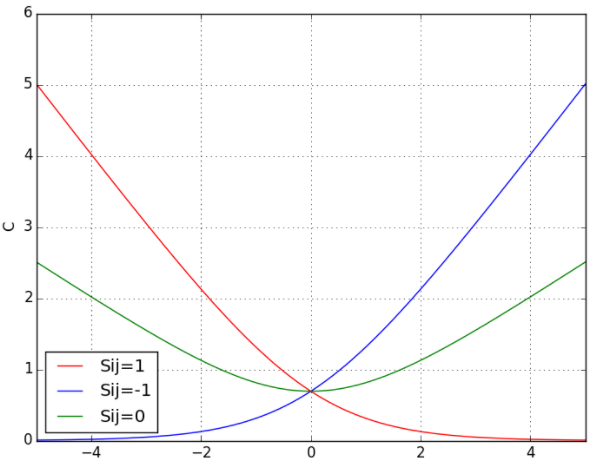• 当两个相关性不同的文档算出来的模型分数相同时，损失函数的值大于0，仍会对这对pair做惩罚，使他们的排序位置区分开。
• 损失函数是一个类线性函数，可以有效减少异常样本数据对模型的影响，因此具有鲁棒性。

$$C=\sum_{(i,j)\in I}C_{ij}$$

#### 合并概率

$$\bar P_{ik}=\frac{\bar P_{ij}\bar P_{jk}}{1+2\bar P_{ij}\bar P_{jk}-\bar P_{ij}-\bar P_{jk}}$$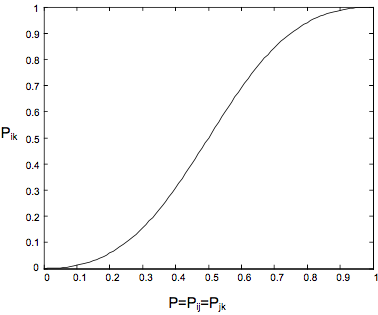• P=0时，有$\bar P_{i,k}=P=0$表示：$D_i$排$D_j$后面,$D_j$排$D_k$的后面，则$D_i$一定排$D_k$的后面；
• 0<P<0.5时，$\bar P_{i,k} < P$；
• P=0.5时，有$\bar P_{i,k} = P = 0.5$表示：$D_i$有一半概率排在有一半概率排在$D_j$前面，$D_j$也有一半概率排在也有一半概率排在$D_k$的前面，则$D_i$同样也是一半的概率排在同样也是一半的概率排在$D_k$的前面；
• 5<P<1时，$\bar p_{i,k}>P$；
• P=1时，有$\bar P_{i,k}=P=1$表示：$D_i$排在$D_j$前面，$D_j$排在$D_k$的前面，则$D_i$也一定排在$D_k$的前面；

$$w_k \rightarrow w_k – \eta \frac{\partial C}{\partial w_k}$$

$\eta$为步长，代价C沿负梯度方向变化。

$$\Delta =\sum_k \frac{\partial C}{\partial w_k} \delta w_k = \sum_k\frac{\partial C}{\partial w_k}(\eta \frac{\partial C}{\partial w_k})=-\eta \sum_k (\frac{\partial C}{\partial w_k})^2<0$$

\begin{align*} \frac{\partial C}{\partial w_k} &= \frac{\partial C}{\partial s_i} \frac{\partial s_i}{\partial w_k} + \frac{\partial C}{\partial s_j} \frac{\partial s_j}{\partial w_k} \\ &= \sigma (\frac{1}{2}(1-S_{ij})-\frac{1}{1 + e^{\sigma (s_i – s_j)}})(\frac{\partial s_i}{\partial w_k}-\frac{\partial s_j}{\partial w_k}) \\ &=\lambda_{ij}(\frac{\partial s_i}{\partial w_k}-\frac{\partial s_j}{\partial w_k}) \\ \end{align*}

$$\lambda_{ij}=\frac{\partial C(s_i-s_j)}{\partial s_i} = \sigma (\frac{1}{2}(1-S_{ij})-\frac{1}{1+e^{\sigma (s_i-s_j)}})$$

#### 加速RankNet训练过程

$$\frac{\partial C}{\partial w_k}=\sum_{(i ,j) \in I}(\frac{\partial C_{ij}}{\partial s_i} \frac{\partial s_i}{\partial w_k} + \frac{\partial C_{ij}}{\partial s_j} \frac{\partial s_j}{\partial w_k})$$

$$\frac{\partial C_{ij}}{\partial s_i}=\sigma (\frac{1}{2}(1-S_{ij})-\frac{1}{1+e^{\sigma (s_i-s_j)}})=-\frac{\partial C_{ij}}{\partial s_j}$$

$$\lambda_{ij}=\frac{\partial C_{ij}}{\partial s_i} = \sigma(\frac{1}{2}(1-S_{ij})-\frac{1}{1+e^{\sigma (s_i-s_j)}})$$

\begin{align*} \frac{\partial C}{\partial w_k} &= \sum_{(i,j) \in I}\sigma (\frac{1}{2}(1-S_{ij})-\frac{1}{1+e^{\sigma (s_i-s_j)}})(\frac{\partial s_i}{\partial w_k}-\frac{\partial s_j}{\partial w_k}) \\ &=\sum_{(i,j) \in I} \lambda_{ij}(\frac{\partial s_i}{\partial w_k}-\frac{\partial s_j}{\partial w_k})\\ &=\sum_i \lambda_i \frac{\partial s_i}{\partial w_k} \\ \end{align*}

$$\lambda_i=\sum_{j:(i,j)\in I}\lambda_{ij}-\sum_{j:(j,i)\in I}\lambda_{ij}$$

$$\frac{\partial C}{\partial w_k}=(\lambda_{12} \frac{\partial s_1}{\partial w_k}-\lambda_{12}\frac{\partial s_2}{\partial w_k})+(\lambda_{13}\frac{\partial s_1}{\partial w_k}-\lambda_{13}\frac{\partial s_3}{\partial w_k})+(\lambda_{23}\frac{\partial s_2}{\partial w_k}-\lambda_{23}\frac{\partial s_3}{\partial w_k})$$

$$\lambda_i=\sum_{j:(i,j)\in I}\lambda_{ij}-\sum_{k:(k,i)\in I}\lambda_{ki}$$

$\lambda_i$决定着第i个doc在迭代中的移动方向和幅度，真实的排在$U_i$前面的doc越少，排在$U_i$后面的doc越多，那么文档$U_i$向前移动的幅度就越大(实际$\lambda_i$负的越多越向前移动)。这表明每个f下次调序的方向和强度取决于同一Query下可以与其组成relative relevance judgment的“pair对”的其他不同label的文档。

### LambdaRank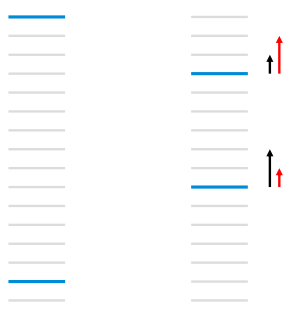$$\lambda_{i j}=\sigma\left[\frac{1}{2}\left(1-S_{i j}\right)-\frac{1}{1+e^{-\sigma\left(s_{i}-s_{j}\right)}}\right], S_{i j}=1$$

$$\lambda_{i j}=-\frac{\sigma}{1+e^{\sigma\left(s_{i}-s_{j}\right)}}\left|\Delta Z_{i j}\right|$$

LambdaRank是一个经验算法，它不是通过显示定义损失函数再求梯度的方式对排序问题进行求解，而是分析排序问题需要的梯度的物理意义，直接定义梯度，即Lambda梯度。

LambdaRank在RankNet的加速算法形式$\lambda_{ij}=\frac{\partial C_{ij}}{\partial s_i} = \sigma(\frac{1}{2}(1-S_{ij})-\frac{1}{1+e^{\sigma (s_i-s_j)}}),S_{ij}=1$的基础上引入评价指标Z（如NDCG、ERR等），把交换两个文档的位置引起的评价指标的变化$|\Delta_{NDCG}|$作为其中一个因子，实验表明对模型效果有显著的提升：

$$\lambda_{ij}=\frac{\partial C(s_i-s_j)}{\partial s_i}=\frac{-\sigma}{1+e^{\sigma (s_i-s_j)}}|\Delta_{NDCG}|$$

### LambdaMART

LambdaMART是LambdaRank的提升树版本，LambdaMART本质上也是属于pairwise排序算法，只不过引入Lambda梯度后，还显示的考察了列表级的排序指标，如NDCG等，因此，也可以算作是listwise的排序算法。LambdaMART由微软于2010年的论文Adapting Boosting for Information Retrieval Measures提出。

LambdaMART是基于 LambdaRank 算法和 MART (Multiple Additive Regression Tree) 算法，将排序问题转化为回归决策树问题。MART 实际就是梯度提升决策树（GBDT, Gradient Boosting Decision Tree）算法。GBDT 的核心思想是在不断的迭代中，新一轮迭代产生的回归决策树模型拟合损失函数的梯度，最终将所有的回归决策树叠加得到最终的模型。LambdaMART 使用一个特殊的 Lambda 值来代替上述梯度，也就是将 LambdaRank 算法与 MART 算法结合起来，是一种能够支持非平滑损失函数的学习排序算法。

MART又称作GBDT，是是一种 Boosting 思想下的算法框架。它通过加法模型，将每次迭代得到的子模型叠加起来；而每次迭代拟合的对象都是学习率与损失函数梯度的乘积。这两点保证了 MART 是一个正确而有效的算法。MART 中最重要的思想，就是每次拟合的对象是损失函数的梯度。值得注意的是，在这里，MART 并不对损失函数的形式做具体规定。实际上，损失函数几乎只需要满足可导这一条件就可以了。这一点非常重要，意味着我们可以把任何合理的可导函数安插在 MART 模型中。LambdaMART 就是用LambdaRank中提出的Lambda梯度代替了损失函数的梯度，将 Lambda和MART结合起来罢了。

• Mart定义了一个框架，缺少一个梯度。
• LambdaRank重新定义了梯度，赋予了梯度新的物理意义。

MART的原理是直接在函数空间对函数进行求解，模型结果由许多棵树组成，每棵树的拟合目标是损失函数的梯度，在LambdaMART中就是Lambda。LambdaMART的具体算法过程如下：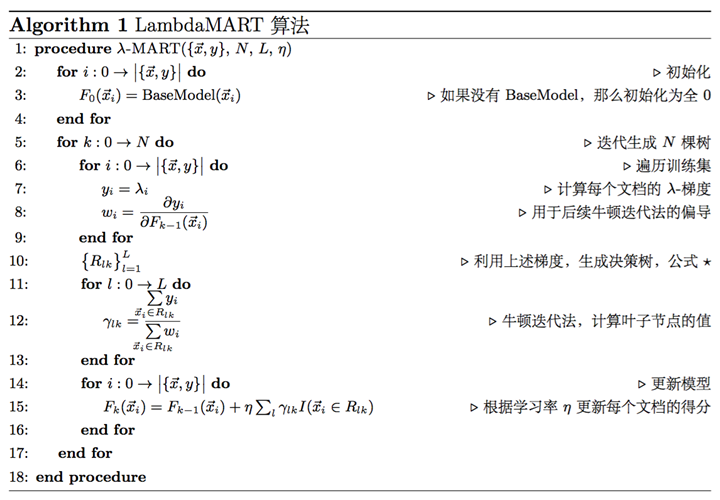MART支持“热启动”，即可以在已经训练好的模型基础上继续训练，在刚开始的时候通过初始化加载进来即可。

1) 每棵树的训练会先遍历所有的训练数据（label不同的文档pair），计算每个pair互换位置导致的指标变化$|\Delta Z_{ij}|$以及Lambda，即$\lambda_{ij}=-\frac{1}{1+e^{s_i-s_j}}|\Delta Z_{ij}|$，然后计算每个文档的Lambda：$\lambda_i=\sum_{j:(i,j)\in I}\lambda_{ij}-\sum_{k:(k,i)\in I}\lambda_{ki}$，再计算每个$\lambda_i$ 的导数$w_i$，用于后面的Newton step求解叶子节点的数值。

2) 创建回归树拟合第一步生成的$\lambda_i$，划分树节点的标准是Mean Square Error，生成一颗叶子节点数为L的回归树。

3) 对第二步生成的回归树，计算每个叶子节点的数值，采用Newton step求解，即对落入该叶子节点的文档集，用公式$\frac{\sum_{x_i \in R_{lm}}y_i}{\sum_{x_i \in R_{lm}}w_i}$计算该叶子节点的输出值。

4) 更新模型，将当前学习到的回归树加入到已有的模型中，用学习率v（也叫shrinkage系数）做regularization。

LambdaMART具有很多优势：

• 适用于排序场景：不是传统的通过分类或者回归的方法求解排序问题，而是直接求解
• 损失函数可导：通过损失函数的转换，将类似于NDCG这种无法求导的IR评价指标转换成可以求导的函数，并且赋予了梯度的实际物理意义，数学解释非常漂亮
• 增量学习：由于每次训练可以在已有的模型上继续训练，因此适合于增量学习
• 组合特征：因为采用树模型，因此可以学到不同特征组合情况
• 特征选择：因为是基于MART模型，因此也具有MART的优势，可以学到每个特征的重要性，可以做特征选择
• 适用于正负样本比例失衡的数据：因为模型的训练对象具有不同label的文档pair，而不是预测每个文档的label，因此对正负样本比例失衡不敏感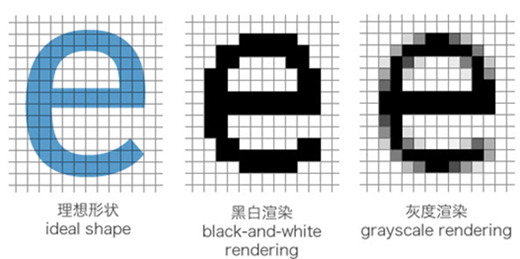##### 网站与APP开发中的字体设置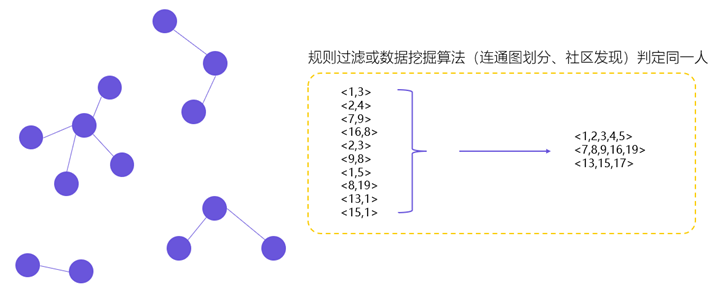##### 用户体系搭建之ID-Mapping